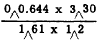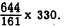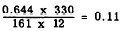Multiplication and Division - Page 9fCustom SearchMULTIPLICATION AND DIVISION COMBINED In problems such asit is generally best to determine the position of the decimal point by means of the method of approximation and to determine the significant digit sequence from the slide rule. Such problems are usually solved by dividing and multi- plying alternately throughout the problem. That is, we divide 0.644 by 161, multiply the quotient by 330, and divide that product by 12. Shifting decimal points, we haveSince there is a combined shift of three places to the left in the divisor, there must also be a combined shift of three places to the left in the dividend. Approximation:= 0.06 x 2 = 0.12 . The step-by-step process of determining the significant digit sequence of this problem is as follows: 1. Place the hairline over 644 on the D scale. 2. Draw the slide so that 161 of the C scale lies under the hairline opposite 644. 3. Opposite the C scale index (on the D scale) is the quotient of 644 + 161. This is to be multiplied by 330, but 330 projects beyond the rule so the C scale indices must be shifted. 4. After shifting the indices, find 330 on the C scale and place the hairline over it. Opposite 330 under the hairline on the D scale is the product of. 5. Next, move the C scale until 12 is under the hairline. Opposite the C scale index (on the D scale) is the final quotient. The digit sequence is 110. The decimal point is then placed according to our approximation: 0.11. Thus,Practice problems. Solve the following problems, using a slide rule:Answers: 1. 10.2 2. 0.817  3. 0.529 SQUARES Squares of numbers are found by reference to the A scale. The numbers on the A scale are the squares of those on the D Scale. The A scale is really a double scale, each division being one-half as large as the corresponding division on the D scale. The use of a double scale for squaring is based upon the fact that the logarithm of the square of a number is twice as large as the logarithm of the number itself. In other words, log N2 = 2 log N This is reasonable, since log N2 = log (N x N)             = log N + log N For a numerical example, suppose that we seek to square 2 by means of logarithms. log 2 = 0.301 log 22 = 2 log 2     = 2 x 0.301     = 0.602 Since each part of the A scale is half as large as the corresponding part of the D scale, the logarithm 0.602 on the A scale will be the same length as the logarithm 0.301 on the D scale. That is, these logarithms will be opposite on the A and D scales. On the A scale as on the D scale, the numbers are written rather than their logarithms. Select several numbers on the D scale, such as 2, 4, 8, 11, and read their squares on the A scale, namely 4, 16, 64, 121. Notice also that the same relation exists for the B and C scales as for the A and D scales. Of interest, also, is the fact that since the A and B scales are made up as are the C and D scales, they too could be used for multiplying or dividing. Placing the Decimal Point Usually the decimal may be placed by the method of approximation. However, close observation will reveal certain facts that eliminate the need for approximations in squaring numbers. Two rules suffice for squaring whole or mixed numbers, as follows: 1. When the square of a number is read on the left half of the A scale, that number will contain twice the number of digits to the left of the decimal point in the original number, less 1.   2. When the square of a number is read on the right half of the A scale, that number will contain twice the number of digits to the left of the decimal point in the original number. EXAMPLE: Square 2.5. SOLUTION: Place the hairline over 25 on the D scale. Read the digit sequence, 625, under the hairline in the left half of the A scale. By rule 1: (2xnumberof digits)-1 = 2(1)-1=1. There is one digit to the left of the decimal point. Thus,     (2.5)2 = 6.25 EXAMPLE: Square 6,340. SOLUTION :     Digit sequence, right half A scale: 402.     By rule 2: 2 x number of digits = 2 x 4 = 8 (digits in answer). Thus,         (6,340)2 = 40,200,OOO Positive Numbers Less Than One   If positive numbers less than one are to be squared, a slightly different version of the preceding rules must be employed. Count the zeros between the decimal point and the first nonzero digit. Consider this count negative. Then the number of zeros between the decimal point and the first significant digit of the squared number may be found as follows: 1. Left half A scale: Multiply the zeros counted by 2 and subtract 1. 2. Right half A scale: Multiply the zeros counted by 2. EXAMPLE : Square 0.0045 SOLUTION:   Digit sequence, right half A scale: 2025. By rule 2: 2(-2) = -4. (Thus, 4 zeros between the decimal point and the first digit.) (0.0045)2= 0.09002025 EXAMPLE: Square 0.0215 SOLUTION:     Digit sequence, left half A scale: 462. By rule 1: 2(-1) -1 = -3         (0.0215)2 = 0.000462Integrated Publishing, Inc. - A (SDVOSB) Service Disabled Veteran Owned Small Business